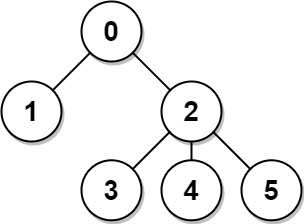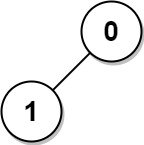## Algorithm

Problem Name: 834. Sum of Distances in Tree

There is an undirected connected tree with `n` nodes labeled from `0` to `n - 1` and `n - 1` edges.

You are given the integer `n` and the array `edges` where `edges[i] = [ai, bi]` indicates that there is an edge between nodes `ai` and `bi` in the tree.

Return an array `answer` of length `n` where `answer[i]` is the sum of the distances between the `ith` node in the tree and all other nodes.

Example 1:```Input: n = 6, edges = [[0,1],[0,2],[2,3],[2,4],[2,5]]
Output: [8,12,6,10,10,10]
Explanation: The tree is shown above.
We can see that dist(0,1) + dist(0,2) + dist(0,3) + dist(0,4) + dist(0,5)
equals 1 + 1 + 2 + 2 + 2 = 8.
Hence, answer = 8, and so on.
```

Example 2:```Input: n = 1, edges = []
Output: 
```

Example 3:```Input: n = 2, edges = [[1,0]]
Output: [1,1]
```

Constraints:

• `1 <= n <= 3 * 104`
• `edges.length == n - 1`
• `edges[i].length == 2`
• `0 <= ai, bi < n`
• `ai != bi`
• The given input represents a valid tree.

## Code Examples

### #1 Code Example with C++ Programming

```Code - C++ Programming```

``````
class Solution {
public:
vector sumOfDistancesInTree(int N, vector>& edges) {
vector>g(N, vector());
for(auto& e: edges){
g[e].push_back(e);
g[e].push_back(e);
}
vectorres(N), child(N), visited(N);
dfs(g, res, child, 0, 0, visited);
for(auto& x: visited) x = 0;
dfs(g, res, child, 0, visited, N);
return res;
}
// Sum of the distances of node 0
void dfs(vector>& g, vector& res, vector& child, int len, int root, vector& visited){
visited[root] = 1;
res += len++;
for(auto& x: g[root]){
if(visited[x]) continue;
dfs(g, res, child, len, x, visited);
child[root] += child[x];
}
child[root] += 1;
}
// Sum of the distances of node 1 to N - 1
void dfs(vector>& g, vector& res, vector& child, int root, vector& visited, int N){
visited[root] = 1;
for(auto& x: g[root]){
if(visited[x]) continue;
res[x] = res[root] - child[x] + N - child[x];
dfs(g, res, child, x, visited, N);
}
}
};
``````
Copy The Code &

Input

cmd
n = 6, edges = [[0,1],[0,2],[2,3],[2,4],[2,5]]

Output

cmd
[8,12,6,10,10,10]

### #2 Code Example with Javascript Programming

```Code - Javascript Programming```

``````
const sumOfDistancesInTree = function (N, edges) {
const graph = createGraph(N, edges)
const counts = new Array(N).fill(0)
const dists = new Array(N).fill(0)
dists = getCount(graph, 0, -1, counts).sum
return transferDist(N, graph, 0, -1, counts, dists)
}

function transferDist(N, graph, u, pre, counts, dists) {
if (pre >= 0) {
const nRight = counts[u]
const nLeft = N - nRight
dists[u] = dists[pre] - nRight + nLeft
}
for (const v of graph[u]) {
if (v !== pre) {
transferDist(N, graph, v, u, counts, dists)
}
}
return dists
}

function getCount(graph, u, pre, counts) {
const output = { nNodes: 0, sum: 0 }
for (const v of graph[u]) {
if (v !== pre) {
const result = getCount(graph, v, u, counts)
output.nNodes += result.nNodes
output.sum += result.nNodes + result.sum
}
}
output.nNodes += 1
counts[u] = output.nNodes
return output
}

function createGraph(N, edges) {
const graph = new Array(N).fill(null).map(() => [])
for (const [u, v] of edges) {
graph[u].push(v)
graph[v].push(u)
}
return graph
}
``````
Copy The Code &

Input

cmd
n = 6, edges = [[0,1],[0,2],[2,3],[2,4],[2,5]]

Output

cmd
[8,12,6,10,10,10]

### #3 Code Example with Python Programming

```Code - Python Programming```

``````
class Solution:
def sumOfDistancesInTree(self, N, edges):
tree = collections.defaultdict(set)
res =  * N
count =  * N
for i, j in edges:

def dfs(root, pre):
for i in tree[root]:
if i != pre:
dfs(i, root)
count[root] += count[i]
res[root] += res[i] + count[i]

def dfs2(root, pre):
for i in tree[root]:
if i != pre:
res[i] = res[root] - count[i] + N - count[i]
dfs2(i, root)
dfs(0, -1)
dfs2(0, -1)
return res
``````
Copy The Code &

Input

cmd
n = 1, edges = []

Output

cmd